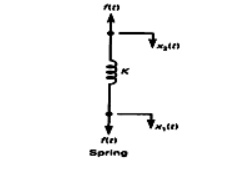Home | | Control System Engineering | Mechanical Translational systems

# Mechanical Translational systems

The model of mechanical translational systems can obtain by using three basic elements mass, spring and dashpot. Force balance equations of idealized elements.

Mechanical Translational systems

The model of mechanical translational systems can obtain by using three basic elements mass, spring and dashpot. When a force is applied to a translational mechanical system, it is opposed by opposing forces due to mass, friction and elasticity of the system. The force acting on a mechanical body is governed by Newton‗s second law of motion. For translational systems it states that the sum of forces acting on a body is zero.

## Force balance equations of idealized elements:

1.

Consider an ideal mass element shown in fig. which has negligible friction and elasticity. Let a force be applied on it. The mass will offer an opposing force which is proportional to acceleration of a body.Let f = applied force

fm =opposing force due to mass Here

fm α M d2 x / dt2

By Newton's second law, f = f m= M d2 x / dt2

2.

Consider an ideal frictional element dash-pot shown in fig. which has negligible mass and elasticity. Let a force be applied on it. The dashpot will be offer an opposing force which is proportional to velocity of the body.Let f = applied force

f b = opposing force due to friction

Here, f b α B dx / dt

By Newton's second law, f = fb = M d x / dt

3.

Consider an ideal elastic element spring is shown in fig. This has negligible mass and friction.Let f = applied force

f k = opposing force due to elasticity

Here, f k α x

By Newtons second law, f = f k = x

Study Material, Lecturing Notes, Assignment, Reference, Wiki description explanation, brief detail
Control Systems : Systems and their Representation : Mechanical Translational systems |